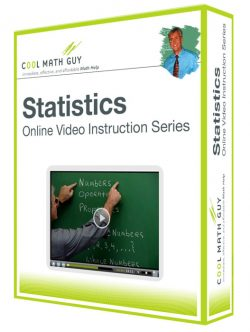Statistics

Course Info

Chapter 1 – GETTING STARTED

• 1.1  What Is Statistics?
• 1.2  Random Samples
• 1.3  Introduction to Experimental Design

Chapter 2 – ORGANIZING DATA

• 2.1  Frequency Distributions, Histograms, and Related Topics
• 2.2  Bar Graphs, Circle Graphs, and Time-Series Graphs
• 2.3  Stem-and-Leaf Displays

Chapter 3 – FRACTIONS

• 3.1  Measures of Central Tendency: Mode, Median, and Mean
• 3.2  Measures of Variation
• 3.3  Percentiles and Box-and-Whisker Plots

Chapter 4 – CORRELATION AND REGRESSION

• 4.1  Scatter Diagrams and Linear Correlation
• 4.2  Linear Regression and the Coefficient of Determination

Chapter 5 – ELEMENTARY PROBABILITY THEORY

• 5.1  What Is Probability
• 5.2  Some Probability Rules – Compound Events
• 5.3  Tree Diagrams and Counting Techniques

Chapter 6 – THE BINOMIAL PROBABILITY DISTRIBUTION AND RELATED TOPICS

• 6.1  Introduction to Random Variables and Probability Distributions
• 6.2  Binomial Probabilities
• 6.3  Additional Properties of the Binomial Distribution

Chapter 7 – NORMAL CURVES AND SAMPLING DISTRIBUTIONS

• 7.1  Graphs of Normal Probability Distributions
• 7.2  Standard Units and Areas Under the Standard Normal Distribution
• 7.3  Areas Under Any Normal Curve
• 7.4  Sampling Distributions
• 7.5  The Central Limit Theorem
• 7.6  Normal Approximations to the Binomial Distribution

Chapter 8 – ESTIMATION

• 8.1  Estimating μ When σ Is Known
• 8.2  Estimating μ When σ Is Unknown
• 8.3  Estimating p in the Binomial Distribution

Chapter 9 – HYPOTHESIS TESTING

• 9.1  Introduction to Statistical Tests
• 9.2  Testing the Mean μ
• 9.3  Testing a Proportion p

Chapter 10 – INFERENCES ABOUT DIFFERENCES

• 10.1  Tests Involving Paired Differences (Dependent Samples)
• 10.2  Inferences About the Difference of Two Means μ1 – μ2
• 10.3  Inferences About the Difference of Two Proportions p1 – p2

Chapter 11 – ADDITIONAL TOPICS USING INFERENCE

• 11.1  Chi-Square: Tests of Independence and of Homogeneity
• 11.2  Chi-Square: Goodness of Fit
• 11.3  Testing a Single Variance or Standard Deviation
• 11.4  Inferences for Correlation and Regression

Course Access Options

•Select options (function(\$) { \$( '.products .post-2520 a' ).click( function() { if ( true === \$(this).hasClass( 'add_to_cart_button' ) ) { return; } ga( 'ec:addProduct', { 'id': '2520', 'name': 'Statistics - Full Course', 'category': 'Statistics/Full Courses', 'position': '1' }); ga( 'ec:setAction', 'click', { list: 'Product List' }); ga( 'send', 'event', 'UX', 'click', ' Product List' ); }); })(jQuery);

Statistics – Full Course

From: \$19.95 / month with 1 day free trial
•Add to shopping bag (function(\$) { \$( '.products .post-2671 a' ).click( function() { if ( true === \$(this).hasClass( 'add_to_cart_button' ) ) { return; } ga( 'ec:addProduct', { 'id': '2671', 'name': 'Statistics - Educators Rate', 'category': 'Statistics/Educators Courses', 'position': '2' }); ga( 'ec:setAction', 'click', { list: 'Product List' }); ga( 'send', 'event', 'UX', 'click', ' Product List' ); }); })(jQuery);

\$50.00